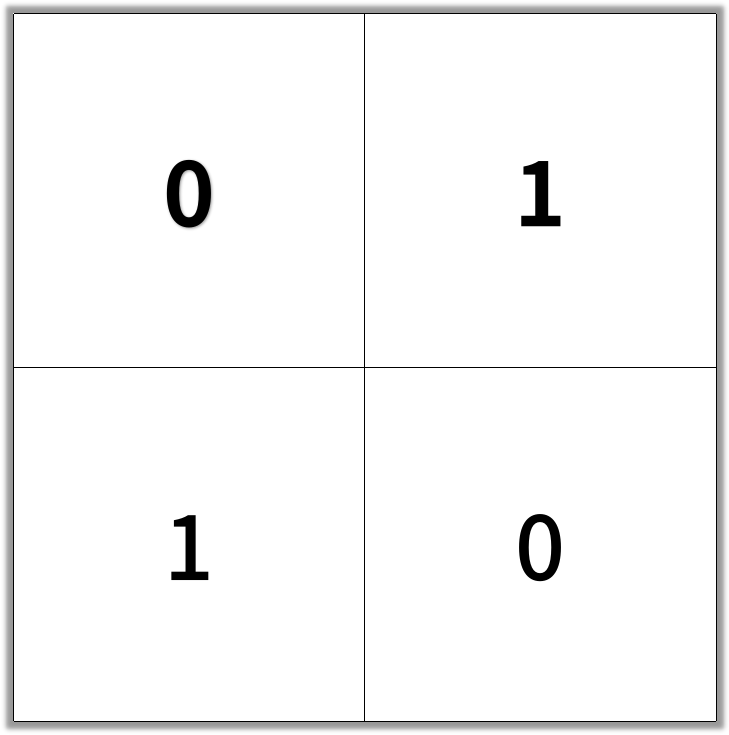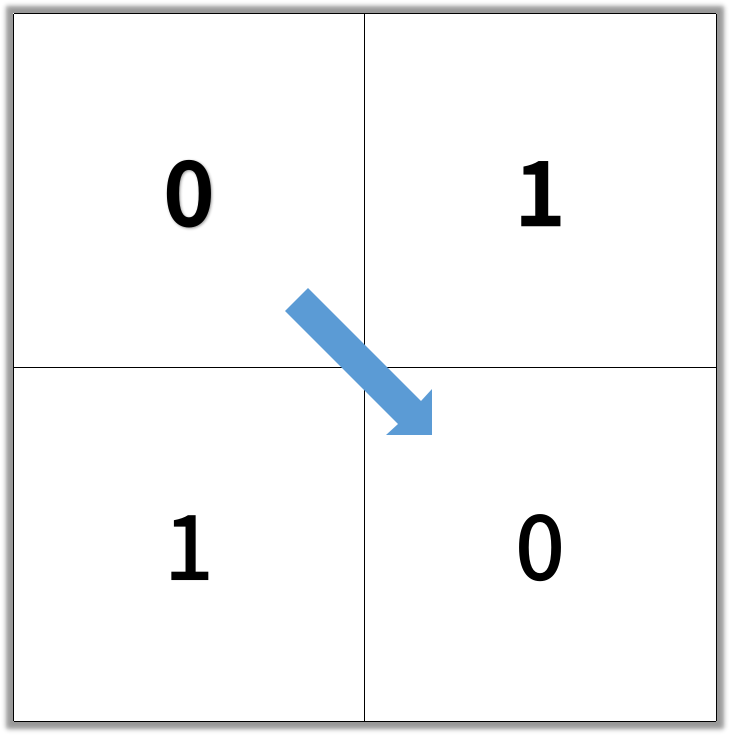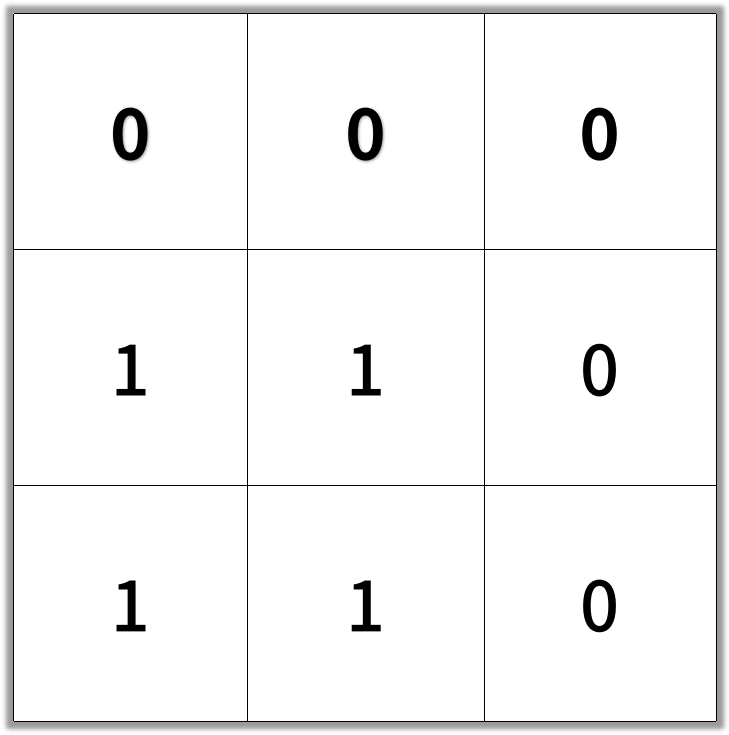## The challenge#

In an N by N square grid, each cell is either empty (0) or blocked (1).

clear path from top-left to bottom-right has length `k` if and only if it is composed of cells `C_1, C_2, ..., C_k` such that:

• Adjacent cells `C_i` and `C_{i+1}` are connected 8-directionally (ie., they are different and share an edge or corner)
• `C_1` is at location `(0, 0)` (ie. has value `grid`)
• `C_k` is at location `(N-1, N-1)` (ie. has value `grid[N-1][N-1]`)
• If `C_i` is located at `(r, c)`, then `grid[r][c]` is empty (ie. `grid[r][c] ==&nbsp;0`).

Return the length of the shortest such clear path from top-left to bottom-right.  If such a path does not exist, return -1.

Example 1:

```Input: [[0,1],[1,0]]Output: 2```

Example 2:

```Input: [[0,0,0],[1,1,0],[1,1,0]]Output: 4```

Note:

1. `1 <= grid.length == grid.length <= 100`
2. `grid[r][c]` is `` or `1`

## The solution#

``````def shortestPathBinaryMatrix(self, grid: List[List[int]]) -> int:
"""
:type grid: List[List[int]]
:rtype: int
"""

if grid != 0:
return -1

q = [[0, 0, 1]]
grid = 1

while len(q) != 0:
# print(q)
k, m, d = q.pop(0)

# grid[k][m] = 1
if k == m == len(grid) - 1:
return d

# UP
if k - 1 >= 0 and grid[k - 1][m] == 0:
q.append([k - 1, m, d + 1])
grid[k-1][m] = 1

# DOWN
if k + 1 < len(grid) and grid[k + 1][m] == 0:
q.append([k + 1, m, d + 1])
grid[k+1][m] = 1

# LEFT
if m - 1 >= 0 and grid[k][m - 1] == 0:
q.append([k, m - 1, d + 1])
grid[k][m-1] = 1

# RIGHT
if m + 1 < len(grid) and grid[k][m + 1] == 0:
q.append([k, m + 1, d + 1])
grid[k][m+1] = 1

# TOP LEFT
if k - 1 >= 0 and m - 1 >= 0 and grid[k - 1][m - 1] == 0:
q.append([k - 1, m - 1, d + 1])
grid[k-1][m-1] = 1

# TOP RIGHT
if k - 1 >= 0 and m + 1 < len(grid) and grid[k - 1][m + 1] == 0:
q.append([k - 1, m + 1, d + 1])
grid[k-1][m+1] = 1

# BOTTOM LEFT
if k + 1 < len(grid) and m - 1 >= 0 and grid[k + 1][m - 1] == 0:
q.append([k + 1, m - 1, d + 1])
grid[k+1][m-1] = 1

# BOTTOM RIGHT
if k + 1 < len(grid) and m + 1 < len(grid) and grid[k + 1][m + 1] == 0:
q.append([k + 1, m + 1, d + 1])
grid[k+1][m+1] = 1

return -1
``````